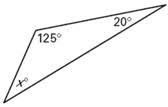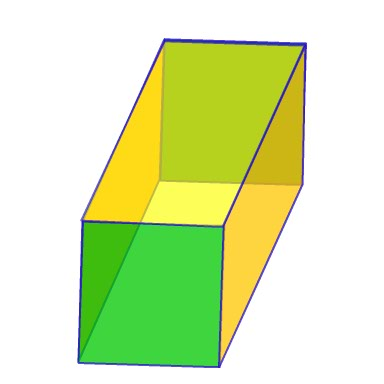# Third Term Examination Mathematics Primary 5 (Basic 5) – Exam Questions

MATHEMATICS

THIRD TERM EXAMINATION

PRIMARY 5 (BASIC 5)

SECTION A

Attempt all the questions.

Choose the correct answer from the options.

1. ______________ is the degree of hotness or coldness of the body.

[a] Power

[b] Temperature

[c] Celsius

2. Convert 30º C to degree Fahrenheit.

[a] 86º

[b] 76º

[c] 66º

3. Each of the angles of an equilateral triangle measure ______________.

[a] 80º

[b] 60º

[c] 45º

4. Which of the plane shapes has four lines of symmetry?

[a] Rectangle

[b] Square

[c] Triangle

5. Two lines are said to be ______________ if they intersect at a right angle.

[a] parallel lines

[b] perpendicular lines

[c] diagonal lines

6. Calculate the lettered angle in the figure below:[a] 55ª

[b] 45ª

[c] 35ª

7. Name these 3 – dimensional shape?[a] Prism

[b] Cylinder

[c] Net cylinder

8. How many edges are there in the shape of a cuboid?

[a] 6 edges

[b] 9 edges

[c] 12 edges

9. The distance round a circle or its boundary called ______________.

[a] diameter

[c] circumference

10. The measurement from base to top is called ______________.

[a] distance

[b] height

[c] perimeter

11. Convert 1001two to base 10?

[a] 910

[b] 1410

[c] 1210

12. Evaluate 11101two – 1011two

[a] 10010two

[b] 10010two

[c] 10011two

13. ______________ means the collection, classification analysis, presentation and interpretation of data.

[a] Graph

[b] Tally

[c] Statistics

Study this data and answer and answer the 2, 2, 2, 4, 8, 5, 6, 4, 3, 4

14. What is the mode of the data?

[a] 2

[b] 5

[c] 5

15. The mean is

[a] 40

[b] 15

[c] 4

Get more Mathematics Exam Questions – Third Term Examination Mathematics Link

SECTION B

Attempt all questions in this section.

QUESTION 1

A. Convert 30º C to degree Fahrenheit.

B. Convert 35º F to degree Celsius.

C. Indicate the type of lines in each case?

QUESTION 2

A. State 2 each properties of the following plane shapes.

I. Rhombus

II. Square

III. Kite

B. Write these angle sizes in degrees:

I. 1½ right angle

II. Two third of a right angle

III. 80% of 3 right angle

QUESTION 3

A. How many edges are there in the following 3 – dimensional shapes:

I. Cylinder

II. Prism

III. A matches box

B. A wheel of circumference 40cm is for 200 times, what was the distance covered?

QUESTION 4

A. Express each of the following in metres:

I. 500 cm

II. 480 cm

III. 10,000 cm

B. Convert 1110two to base 10.

QUESTION 5

A. In a class the number of pupils who passed English is 40, mathematics 90, science 50, and literature 45. Represent this information in a pictogram.

B. Find the simple interest on ₦600.00 for 4 years at 6% per annum.